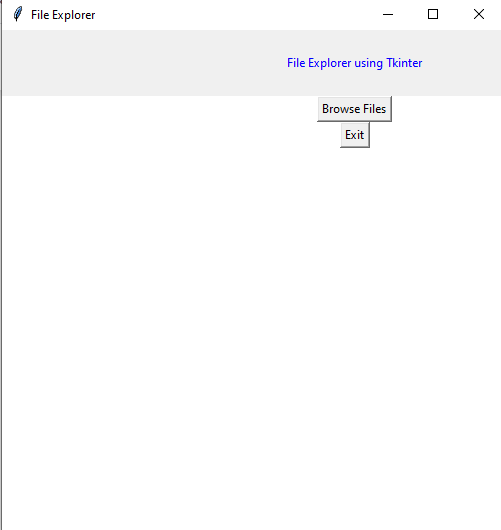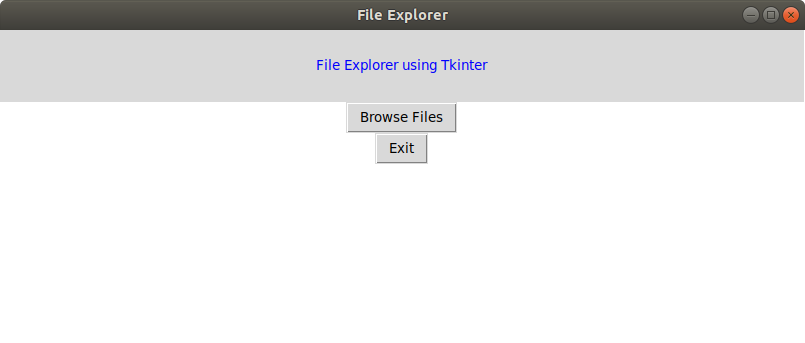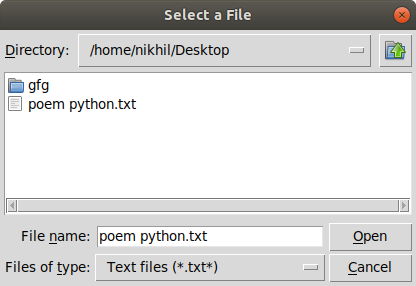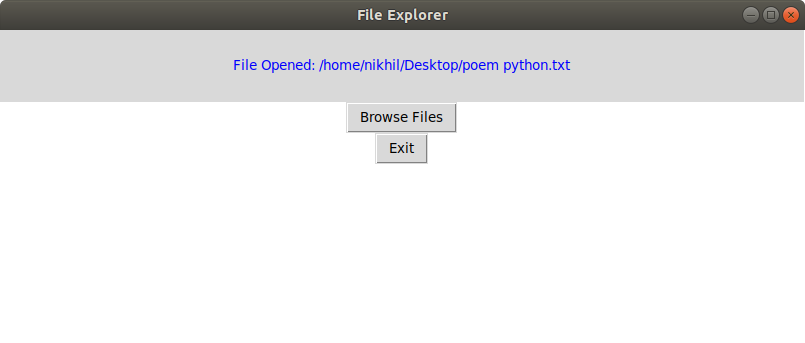# File Explorer in Python using Tkinter

## File Explorer in Python using Tkinter

Python offers various modules to create graphics programs. Out of these Tkinter provides the fastest and easiest way to create GUI applications.

The following steps are involved in creating a tkinter application:

• Importing the Tkinter module.
• Creation of the main window (container).
• Addition of widgets to the main window
• Applying the event Trigger on widgets like buttons, etc.

The GUI would look like below:#### Creating the File Explorer

In order to do so, we have to import the filedialog module from Tkinter. The File dialog module will help you open, save files or directories.
In order to open a file explorer, we have to use the method, askopenfilename(). This function creates a file dialog object.

Syntax: tkFileDialog.askopenfilename(initialdir = “/”,title = “Select file”,filetypes = ((“file_type”,”*.extension”),(“all files”,”*.*”)))
Parameters:

1. initialdir: We have to specify the path of the folder that is to be opened when the file explorer pops up.
2. title: The title of file explorer opened.
3. filetypes: Here we can specify different kinds of file extensions so that the user can filter based on different file types

Below is the implementation

 `# Python program to create` `# a file explorer in Tkinter` ` ` `# import all components` `# from the tkinter library` `from` `tkinter ``import` `*` ` ` `# import filedialog module` `from` `tkinter ``import` `filedialog` ` ` `# Function for opening the` `# file explorer window` `def` `browseFiles():` `    ``filename ``=` `filedialog.askopenfilename(initialdir ``=` `"/"``,` `                                          ``title ``=` `"Select a File"``,` `                                          ``filetypes ``=` `((``"Text files"``,` `                                                        ``"*.txt*"``),` `                                                       ``(``"all files"``,` `                                                        ``"*.*"``)))` `     ` `    ``# Change label contents` `    ``label_file_explorer.configure(text``=``"File Opened: "``+``filename)` `     ` `     ` `                                                                                                 ` `# Create the root window` `window ``=` `Tk()` ` ` `# Set window title` `window.title(``'File Explorer'``)` ` ` `# Set window size` `window.geometry(``"500x500"``)` ` ` `#Set window background color` `window.config(background ``=` `"white"``)` ` ` `# Create a File Explorer label` `label_file_explorer ``=` `Label(window,` `                            ``text ``=` `"File Explorer using Tkinter"``,` `                            ``width ``=` `100``, height ``=` `4``,` `                            ``fg ``=` `"blue"``)` ` ` `     ` `button_explore ``=` `Button(window,` `                        ``text ``=` `"Browse Files"``,` `                        ``command ``=` `browseFiles)` ` ` `button_exit ``=` `Button(window,` `                     ``text ``=` `"Exit"``,` `                     ``command ``=` `exit)` ` ` `# Grid method is chosen for placing` `# the widgets at respective positions` `# in a table like structure by` `# specifying rows and columns` `label_file_explorer.grid(column ``=` `1``, row ``=` `1``)` ` ` `button_explore.grid(column ``=` `1``, row ``=` `2``)` ` ` `button_exit.grid(column ``=` `1``,row ``=` `3``)` ` ` `# Let the window wait for any events` `window.mainloop()`

Output:Last Updated on November 13, 2021 by admin

## Python – Iterate over multiple lists simultaneouslyPython – Iterate over multiple lists simultaneously

Python | Iterate over multiple lists simultaneously   Iterating over single lists, refers to using

## Python Bitwise OperatorsPython Bitwise Operators

Python Bitwise Operators Operators are used to perform operations on values and variables. These are the

## Working With JSON Data in PythonWorking With JSON Data in Python

Working With JSON Data in Python Introduction of JSON in Python : The full form

## Python statistics | mean() functionPython statistics | mean() function

Python statistics | mean() function Python is a very popular language when it comes to

## Python – Pandas.to_datetime()Python – Pandas.to_datetime()

Python | Pandas.to_datetime() When a csv file is imported and a Data Frame is made,

## Python – Check if a given object is list or notPython – Check if a given object is list or not

Python – Check if a given object is list or not Given an object, the

## Python program to check if a string is palindrome or notPython program to check if a string is palindrome or not

Python program to check if a string is palindrome or not Given a string, write

## Python ceil() functionPython ceil() function

Python ceil() method The method ceil() in Python returns a ceiling value of x i.e.,